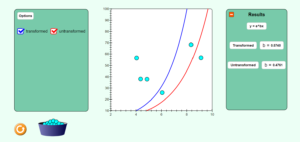# Chapter 06 Transformed vs Untransformed Data Regression Simulation

Description:  This simulation depicts the effect of transforming the data for regression analysis.  Taking a simple model like y=ebx for given y vs x data, we show the effect of choosing the convenience of transforming the data.Keywords:  regression, nonlinear regression, transformation of data, untransformed data

Learning Objectives:  After successful completion of running the simulation with proper questions asked by the instructor, the student would be able to 1) note that transformation of data can give significantly different results for the regression constant 2) how outliers can affect the transformed and untransformed regression models

Full Resources:  The full resources for the topic of the nonlinear regression models are given here which include textbook content, a PowerPoint presentation, a multiple-choice test, audiovisual lectures, and application examples.